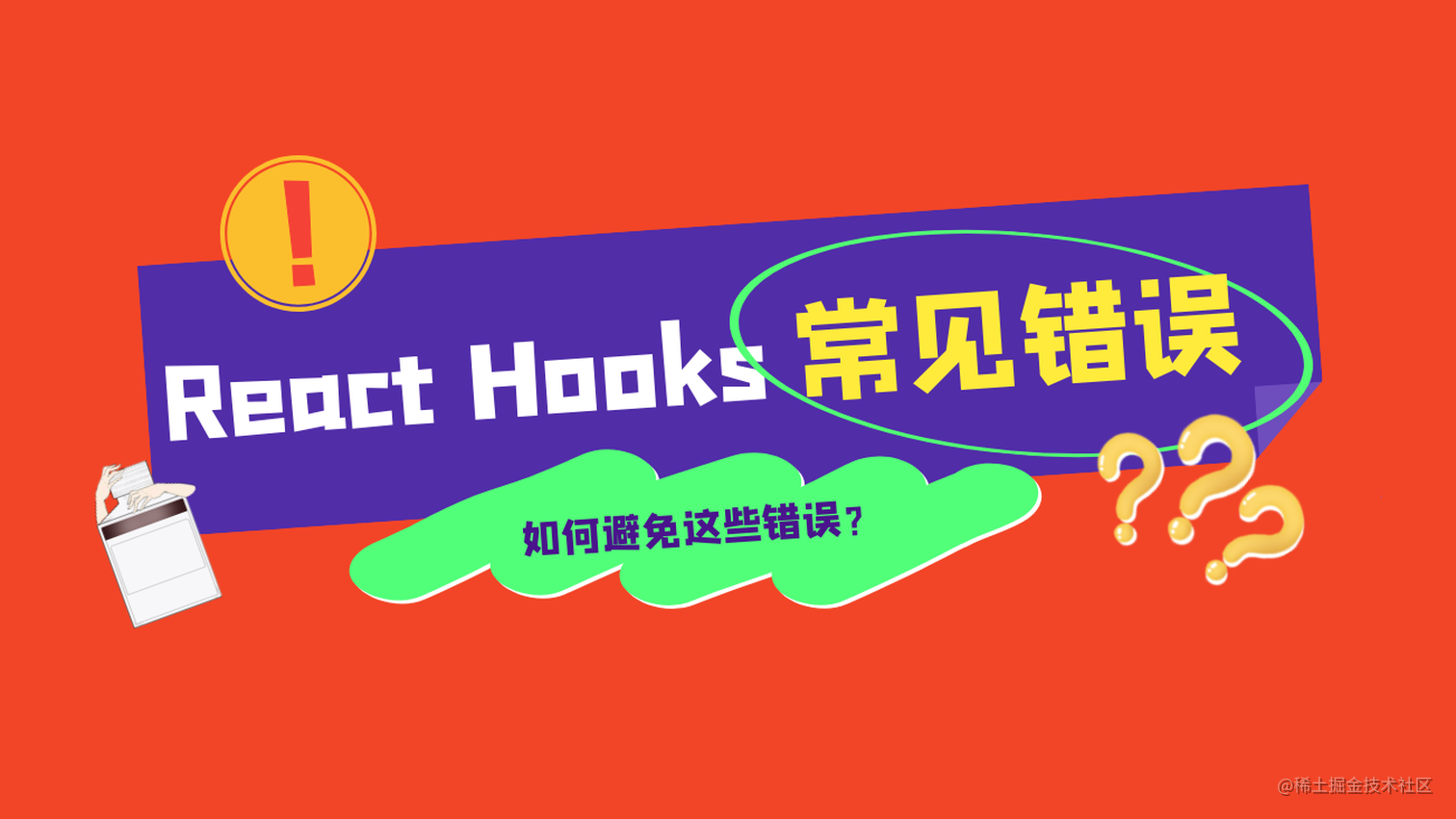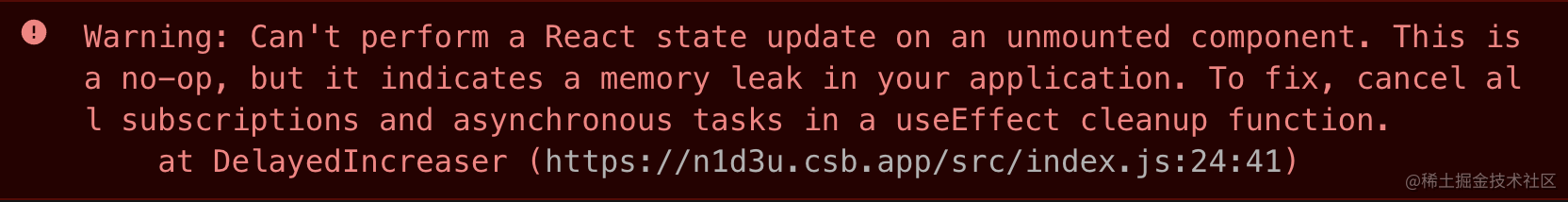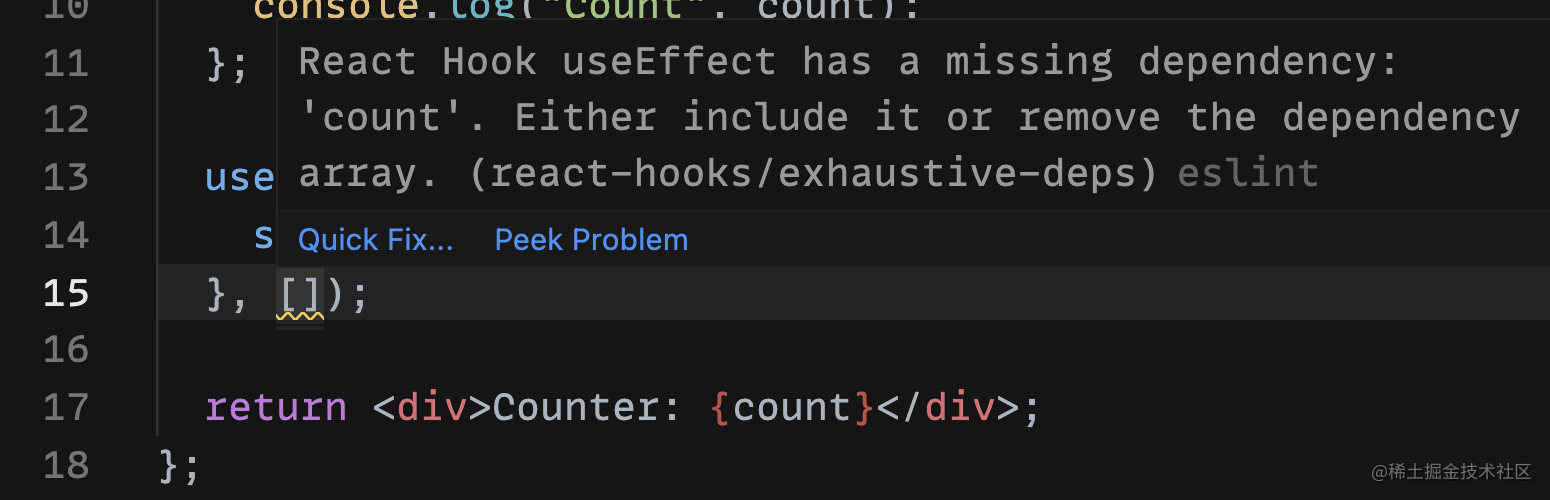# 使用 React Hooks 时要避免的6个错误1. 不要改变 hooks 的调用顺序；
2. 不要使用旧的状态；
3. 不要创建旧的闭包；
4. 不要忘记清理副作用；
5. 不要在不需要重新渲染时使用useState；
6. 不要缺少useEffect依赖。

### 1. 不要改变 hooks 的调用顺序

``````const FetchGame = ({ id }) => {
if (!id) {
return '请选择一个游戏';
}

const [game, setGame] = useState({
name: '',
description: ''
});

useEffect(() => {
const fetchGame = async () => {
const response = await fetch(`/api/game/\${id}`);
const fetchedGame = await response.json();
setGame(fetchedGame);
};
fetchGame();
}, [id]);

return (
<div>
<div>Name: {game.name}</div>
<div>Description: {game.description}</div>
</div>
);
}

``````const FetchGame = ({ id }) => {
const [game, setGame] = useState({
name: '',
description: ''
});

useEffect(() => {
const fetchGame = async () => {
const response = await fetch(`/api/game/\${id}`);
const fetchedGame = await response.json();
setGame(fetchedGame);
};
id && fetchGame();
}, [id]);

if (!id) {
return '请选择一个游戏';
}

return (
<div>
<div>Name: {game.name}</div>
<div>Description: {game.description}</div>
</div>
);
}

React官方文档中的Hook规则：《Hook 规则》，可以使用插件eslint-plugin-react-hooks来帮助我们检查这些规则。

### 2. 不要使用旧的状态

``````const Increaser = () => {
const [count, setCount] = useState(0);

const increase = useCallback(() => {
setCount(count + 1);
}, [count]);

const handleClick = () => {
increase();
increase();
increase();
};

return (
<>
<button onClick={handleClick}>+</button>
<div>Counter: {count}</div>
</>
);
}

``````const handleClick = () => {
setCount(count + 1);
setCount(count + 1);
setCount(count + 1);
};

``````const Increaser = () => {
const [count, setCount] = useState(0);

const increase = useCallback(() => {
setCount(count => count + 1);
}, [count]);

const handleClick = () => {
increase();
increase();
increase();
};

return (
<>
<button onClick={handleClick}>+</button>
<div>Counter: {count}</div>
</>
);
}

``````setValue(prevValue => prevValue + someResult)

### 2. 不要创建旧的闭包

``````useEffect(callback, deps)
useCallback(callback, deps)

``````const WatchCount = () => {
const [count, setCount] = useState(0);

useEffect(() => {
setInterval(function log() {
console.log(`Count: \${count}`);
}, 2000);
}, []);

const handleClick = () => setCount(count => count + 1);

return (
<>
<button onClick={handleClick}>+</button>
<div>Count: {count}</div>
</>
);
}``````const WatchCount = () => {
const [count, setCount] = useState(0);

useEffect(function() {
const id = setInterval(function log() {
console.log(`Count: \${count}`);
}, 2000);
return () => clearInterval(id);
}, [count]);

const handleClick = () => setCount(count => count + 1);

return (
<>
<button onClick={handleClick}>+</button>
<div>Count: {count}</div>
</>
);
}

### 4. 不要忘记清理副作用

``````const DelayedIncreaser = () => {
const [count, setCount] = useState(0);
const [increase, setShouldIncrease] = useState(false);

useEffect(() => {
if (increase) {
setInterval(() => {
setCount(count => count + 1)
}, 1000);
}
}, [increase]);

return (
<>
<button onClick={() => setShouldIncrease(true)}>
+
</button>
<div>Count: {count}</div>
</>
);
}

const MyApp = () => {
const [show, setShow] = useState(true);

return (
<>
{show ? <DelayedIncreaser /> : null}
<button onClick={() => setShow(false)}>卸载</button>
</>
);
}``````useEffect(() => {
if (increase) {
const id = setInterval(() => {
setCount(count => count + 1)
}, 1000);
return () => clearInterval(id);
}
}, [increase]);

### 5. 不要在不需要重新渲染时使用useState

``````const Counter = () => {
const [counter, setCounter] = useState(0);

const onClickCounter = () => {
setCounter(counter => counter + 1);
};

const onClickCounterRequest = () => {
apiCall(counter);
};

return (
<div>
<button onClick={onClickCounter}>Counter</button>
<button onClick={onClickCounterRequest}>Counter Request</button>
</div>
);
}

``````const Counter = () => {
const counter = useRef(0);

const onClickCounter = () => {
counter.current++;
};

const onClickCounterRequest = () => {
apiCall(counter.current);
};

return (
<div>
<button onClick={onClickCounter}>Counter</button>
<button onClick={onClickCounterRequest}>Counter Request</button>
</div>
);
}

### 6. 不要缺少useEffect依赖

useEffect是React Hooks中最常用的Hook之一。默认情况下，它总是在每次重新渲染时运行。但这样就可能会导致不必要的渲染。我们可以通过给useEffect设置依赖数组来避免这些不必要的渲染。 ​

``````const Counter = () => {
const [count, setCount] = useState(0);

const showCount = (count) => {
console.log("Count", count);
};

useEffect(() => {
showCount(count);
}, []);

return (
<div>Counter: {count}</div>
);
}``````const Counter = () => {
const [count, setCount] = useState(0);

const showCount = (count) => {
console.log("Count", count);
};

useEffect(() => {
showCount(count);
}, [count]);

return (
<div>Counter: {count}</div>
);
}

``````useEffect(() => {
showCount(996);
}, []);